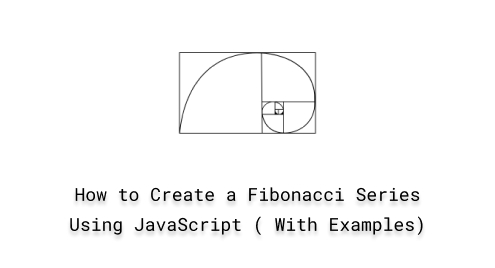# How to Create a Fibonacci Series Using JavaScript (With Examples)

The sequence of Fibonacci numbers is a well-known mathematical series that begins with 0 and 1, and each following number equals the sum of the two preceding ones, as in “0, 1, 1, 2, 3, 5, 8, 13, 21,……“. Fibonacci numbers play a significant role in various algorithms such as the “Fibonacci Search” algorithm and “Dynamic Programming” techniques.

More specifically, it is utilized in graphics algorithms to generate visually pleasing patterns and forms, and it has many exciting qualities that occur naturally in many elements of life, such as plant growth patterns, animal populations, and even financial markets.

## How to Create a Fibonacci Series Using JavaScript?

For creating the Fibonacci series in JavaScript, use the following techniques:

• Iterative method
• Recursive method

### Method 1: Fibonacci Series Using Iterative Method

The most commonly used technique is the “iterative” method that includes “for” loop, “while” loop, “do-while” loop, and so on. Here, we will discuss the “for” loop approach for the Fibonacci series in JavaScript.

Syntax

The provided syntax is utilized for the “for” loop:

```for (initialization; condition; increment/decrement) {
// Code to be executed in each iteration
}```

Example

Here, we will first define a function named “fibonacciSeries()” that takes the number range as “r” which indicates how many terms of the Fibonacci sequence you want to generate. The “for” loop will print the series starting with 0 and 1, and each subsequent number is the sum of the two preceding ones. The function will return a series of Fibonacci numbers up to the specified number range:

```function fibonacciSeries(r) {
let a = 0, b = 1, c;
for (let i = 0; i < r; i++) {
console.log(a);
c = a + b;
a = b;
b = c;
}
}```

Call the function by passing the number range “15” which indicates the series will be of 15 numbers:

`fibonacciSeries(15);`

The detailed code snippet is as follows: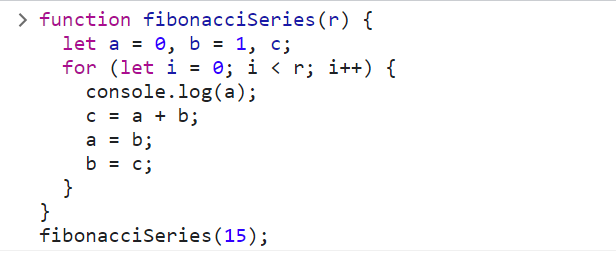Output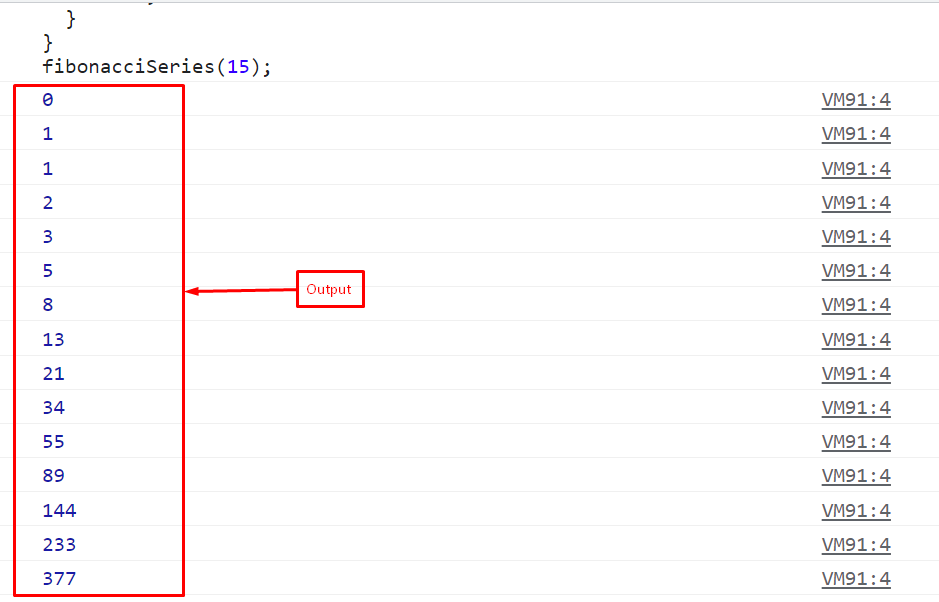Example

If you want to get the Fibonacci series as a list in an array, use the “for” loop with the “push()” method. It gives an array containing the Fibonacci sequence up to the specified number of terms:

```function fibonacciSeries(r) {
const series = [0, 1];
for (let i = 2; i < r; i++) {
const n = series[i - 1] + series[i - 2];
series.push(n);
}
return series;
}```

Call the function by providing a range for Fibonacci numbers up to 10:

`fibonacciSeries(10);`

The output has been successfully displaying the first 10 Fibonacci numbers in an array: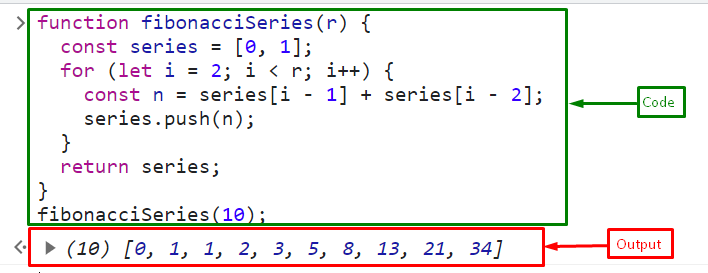### Method 2: Fibonacci Series Using Recursive Method

Another way to create a Fibonacci series is to use the “recursive” approach. In this technique, we will define a function that calls itself to calculate each Fibonacci number. While the recursive approach can be elegant, it may not be the most efficient for large values of numbers due to the repetitive calculations.

Example

In this example, we will generate a Fibonacci sequence using the recursive method where the base conditions are if the number range is equal to 1, print 0, if the number is equal to 2, print 0, and 1. If the number range is more than 2, like 10, then, call the function itself as (number range -1) and store it in a variable “series”. Call the function repeatedly and push the values in the “series”:

```function fibonacciSeries(num) {
let array = [];
if (num == 1) return ;
if (num == 2) return [0, 1];
else {
const series = fibonacciSeries(num -1);
series.push(series[series.length - 1] + series[series.length - 2]);
return series;
}
}```

Here, we will print the first 7 Fibonacci numbers by passing “7” as an argument in function “fibonacciSeries()”:

`fibonacciSeries(7);`

Output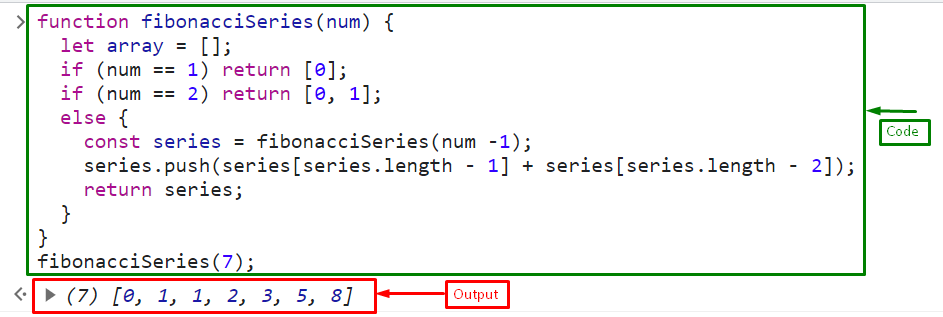If you want to get the specified term of the Fibonacci number series, you have to try this code snippet:

```function fibonacciSeries(num) {
if (num == 1) return 0;
if (num == 2) return 1;
return fibonacciSeries(num - 1) + fibonacciSeries(num - 2);
}```

Here, we want the 5th term of the sequence:

`console.log("The 5th term of the Fibonacci series is: " + fibonacciSeries(5));`

The output prints “3” which is the 5th term of the Fibonacci sequence: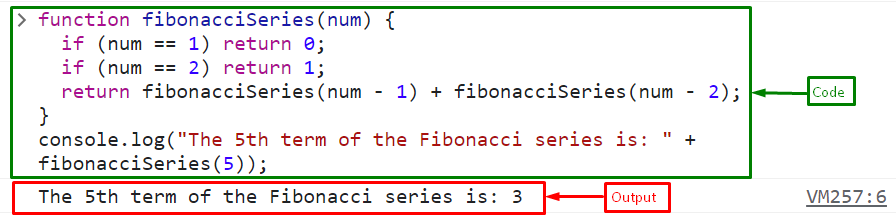That was all about the Fibonacci sequence in JavaScript.

## Conclusion

To create the Fibonacci series in JavaScript utilize the “Iterative” or the “Recursive” approach. The Iterative method is the most commonly used approach while the recursive approach can be elegant, it may not be the most efficient for large values of numbers due to the repetitive calculations. This article described the Fibonacci series in JavaScript.